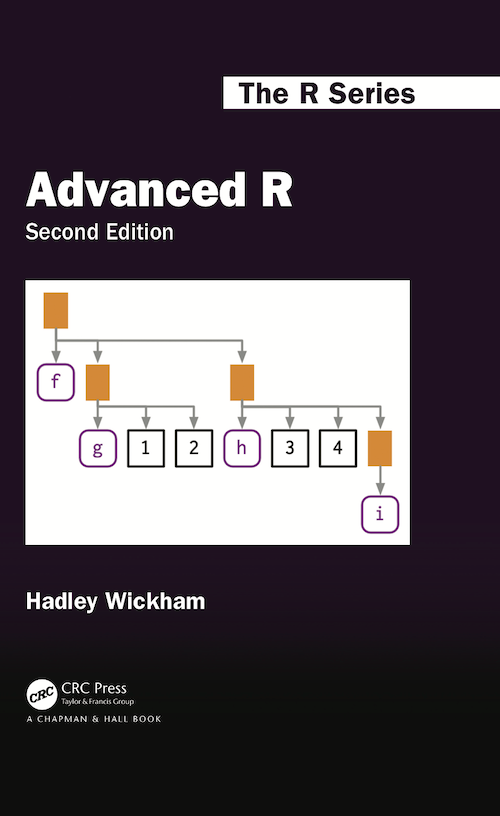knitr::opts_chunk\$set(error = TRUE)
suppressPackageStartupMessages(library(tidyverse))
suppressPackageStartupMessages(library(rlang))
suppressPackageStartupMessages(library(lobstr))
suppressPackageStartupMessages(library(withr))
suppressPackageStartupMessages(library(glue))
# devtools::install_github("openpharma/simaerep@v0.3.1")
suppressPackageStartupMessages(library(simaerep))

Functions

Conventions for arguments that come from a set of strings

center <- function(x, type = c("mean", "median", "trimmed")) {
type <- match.arg(type)
switch(type,
mean = mean(x),
median = median(x),
trimmed = mean(x, trim = .1))
}

Functions to Investigate Functions

.f <- function(x,y){
# Comment
x + y
}
formals(.f) # returns arguments
## \$x
##
##
## \$y
body(.f) # returns body without comments
## {
##     x + y
## }
environment(.f)
## <environment: R_GlobalEnv>
attributes(.f)\$srcref # returns body with commments
## function(x,y){
##   # Comment
##   x + y
## }

Get all Functions from package

objs <- mget(ls("package:base", all = TRUE), inherits = TRUE)
funs <- Filter(is.function, objs)

Function with the most arguments

primitive base functions written in C are either of type “builtin” or “special”. formals(), body()and environment() will return NULL for those functions.

df_funs <- tibble(funs = funs,
names = names(funs) ) %>%
mutate(formals = map(funs, formals),
n_args = map_int(formals, length),
type = map_chr(funs, typeof)) %>%
arrange(desc(n_args))

df_funs
## # A tibble: 1,323 × 5
##    funs         names            formals      n_args type
##    <named list> <chr>            <named list>  <int> <chr>
##  1 <fn>         scan             <pairlist>       22 closure
##  2 <fn>         format.default   <pairlist>       16 closure
##  3 <fn>         source           <pairlist>       16 closure
##  4 <fn>         formatC          <pairlist>       15 closure
##  5 <fn>         library          <pairlist>       13 closure
##  6 <fn>         merge.data.frame <pairlist>       13 closure
##  7 <fn>         prettyNum        <pairlist>       13 closure
##  8 <fn>         system2          <pairlist>       11 closure
##  9 <fn>         print.default    <pairlist>       10 closure
## 10 <fn>         save             <pairlist>       10 closure
## # … with 1,313 more rows
df_funs %>%
group_by(type, n_args == 0) %>%
count()
## # A tibble: 4 × 3
## # Groups:   type, n_args == 0 
##   type    `n_args == 0`     n
##   <chr>   <lgl>         <int>
## 1 builtin TRUE            165
## 2 closure FALSE          1072
## 3 closure TRUE             47
## 4 special TRUE             39

Scoping

Search Environment

my_string <- "hello world"

exists("my_string")
##  TRUE
exists("my_string_2")
##  FALSE
get0("my_string")
##  "hello world"
get0("my_string_2")
## NULL
ls() # get all variable names
##  "center"    "df_funs"   "funs"      "my_string" "objs"

List unbound global variables

g12 <- function() x + 1
codetools::findGlobals(g12)
##  "+" "x"
g13 <- function(x) x + 1
codetools::findGlobals(g13)
##  "+"

Lazy Evaluation of Arguments

arguments even when given as expressions will only be evaluated when called in the function, these structures are called promises. So this works, surprisingly:

h04 <- function(x = 1, y = x * 2, z = a + b) {
a <- 10
b <- 100

c(x, y, z)
}

But only when supplied as default arguments not when user-supplied

h04(1, x * 2, a + b)
## Error in h04(1, x * 2, a + b): object 'x' not found

User-Supplied arguments are evaluated before they are passed to the function, that is why infix functions and operators such as + and %%are working.

Default arguments are evaluated as promises only when they are called within the function.

Reminder infix functions take two arguments, code that comes directly before and right after. User defined infix functions need to be defined like this with `%name% <- function(lhs, rhs)`

x <- 1

!is.null(x) && length(x) == 1 && x > 0
##  TRUE
# in python we would need to put brackets around the logic statements
(!is.null(x)) && (length(x) == 1) && (x > 0)
##  TRUE
c(TRUE, FALSE) && c(TRUE, FALSE) # evaluation stops at first element
##  TRUE
c(TRUE, FALSE) & c(TRUE, FALSE) # all elements are pair-wise evaluated
##   TRUE FALSE
FALSE && NULL # evaluation stops after result is determined by first argument FALSE
##  FALSE
FALSE || NULL # gives error because NULL needs to be evaluated for OR condition
## Error in FALSE || NULL: invalid 'y' type in 'x || y'
FALSE & NULL # I would expect this to error too
## logical(0)
FALSE | NULL # this as well mmh
## logical(0)

Default or User Supplied argument

h06 <- function(x = 10) {
is_default <- missing(x)
return(is_default)
}

h06()
##  TRUE
h06(10)
##  FALSE

Capture dot dot dot

i03 <- function(...) {
list(first = ..1, third = ..3)
}
str(i03(1, 2, 3))
## List of 2
##  \$ first: num 1
##  \$ third: num 3
i04 <- function(...) {
list(...)
}
str(i04(a = 1, b = 2))
## List of 2
##  \$ a: num 1
##  \$ b: num 2

Exit Handler on.exit()

Always set add = TRUE when using on.exit(). If you don’t, each call to on.exit() will overwrite the previous exit handler. Even when only registering a single handler, it’s good practice to set add = TRUE so that you won’t get any unpleasant surprises if you later add more exit handlers.

j06 <- function(x) {
cat("Hello\n")

if (x) {
return(10)
} else {
stop("Error")
}
}

j06(TRUE)
## Hello
## Goodbye!
##  10
j06(FALSE)
## Hello
## Error in j06(FALSE): Error
## Goodbye!

Better than using on.exit is actually to use functions of the withr package that automatically provide cleanups for files and directories

Capture output capture.output()

also captures error messages

Everything that happens in R is a function call

Almost everything can be rewritten as a function call

x + y
`+`(x, y)
names(df) <- c("x", "y", "z")
## Error in names(df) <- c("x", "y", "z"): names() applied to a non-vector
`names<-`(df, c("x", "y", "z"))
## Error in eval(expr, envir, enclos): names() applied to a non-vector
for(i in 1:10) print(i)
##  1
##  2
##  3
##  4
##  5
##  6
##  7
##  8
##  9
##  10
`for`(i, 1:10, print(i))
##  1
##  2
##  3
##  4
##  5
##  6
##  7
##  8
##  9
##  10

Replacement Functions

`second<-` <- function(x, value) {
x <- value
x
}

x <- 1:10
second(x) <- 5

x
##    1  5  3  4  5  6  7  8  9 10

Environments

Generally, an environment is similar to a named list, with four important exceptions:

• Every name must be unique.
• The names in an environment are not ordered.
• An environment has a parent.
• Environments are not copied when modified.
e1 <- env(
a = FALSE,
b = "a",
c = 2.3,
d = 1:3,
)

rlang::env_print(e1)
## <environment: 0x7fd2d346bac0>
## parent: <environment: global>
## bindings:
##  * a: <lgl>
##  * b: <chr>
##  * c: <dbl>
##  * d: <int>
rlang::env_names(e1)
##  "a" "b" "c" "d"

Create a parent environment

e2 <- env(e1, letters = LETTERS)

rlang::env_parent(e2)
## <environment: 0x7fd2d346bac0>
rlang::env_parents(e2)
## []   <env: 0x7fd2d346bac0>
## [] \$ <env: global>

Packages

Composition of the global environment

search()
##   ".GlobalEnv"        "package:simaerep"  "package:glue"
##   "package:withr"     "package:lobstr"    "package:rlang"
##   "package:forcats"   "package:stringr"   "package:dplyr"
##  "package:tibble"    "package:ggplot2"   "package:tidyverse"
##  "package:stats"     "package:graphics"  "package:grDevices"
##  "package:utils"     "package:datasets"  "package:methods"
rlang::search_envs()
##  [] \$ <env: global>
##  [] \$ <env: package:simaerep>
##  [] \$ <env: package:glue>
##  [] \$ <env: package:withr>
##  [] \$ <env: package:lobstr>
##  [] \$ <env: package:rlang>
##  [] \$ <env: package:forcats>
##  [] \$ <env: package:stringr>
##  [] \$ <env: package:dplyr>
## [] \$ <env: package:purrr>
## [] \$ <env: package:tidyr>
## [] \$ <env: package:tibble>
## [] \$ <env: package:ggplot2>
## [] \$ <env: package:tidyverse>
## [] \$ <env: package:stats>
## [] \$ <env: package:graphics>
## [] \$ <env: package:grDevices>
## [] \$ <env: package:utils>
## [] \$ <env: package:datasets>
## ... and 3 more environments

Package Functions

• passively bound by one environment (where they can be called from)
• actively bind one environment (which they use to make their calls)

for regular functions both environments are the same. For functions loaded from packages the bind environment is defined by the package namespace created from the package NAMESPACE file. Like this they are not affected by overrides in the execution environment.

Callstacks

use lobstr::cst() in a similar way to traceback() to visualise the callstack.

f <- function(x) {
g(x = 2)
}
g <- function(x) {
h(x = 3)
}
h <- function(x) {
stop()
}

f(x = 1)
## Error in h(x = 3):
traceback()
## No traceback available
h <- function(x) {
lobstr::cst()
print("do I get executed =")
}

f(x = 1)
##     █
##  1. └─global::f(x = 1)
##  2.   └─global::g(x = 2)
##  3.     └─global::h(x = 3)
##  4.       └─lobstr::cst()
##  "do I get executed ="

Conditions

Warnings

give_warning <- function() {
warning("You have been warned")
}

withr::with_options(list(warn = 1), give_warning()) # causes warning to appear immediately
## Warning in give_warning(): You have been warned
withr::with_options(list(warn = 2), give_warning()) # convert warning to error
## Error in give_warning(): (converted from warning) You have been warned

Condition Objects

conditions such as messages, warnings and errors are objects

cnd <- rlang::catch_cnd(stop("An error"))
str(cnd)
## List of 2
##  \$ message: chr "An error"
##  \$ call   : language force(expr)
##  - attr(*, "class")= chr [1:3] "simpleError" "error" "condition"

tryCatch

tryCatch executes code and has arguments for different types of condition objects. Each arguments takes a function with a single condition object argument.

try_me <- function(expr) {
tryCatch(
error = function(cnd) print("error"),
warning = function(cnd) print("warning"),
message = function(cnd) print("message"),
finally = print("finished"),
expr
)
}

try_me(stop("hello"))
##  "error"
##  "finished"
try_me(warning("hello"))
##  "warning"
##  "finished"
try_me(message("hello"))
##  "message"
##  "finished"

variable assignments within trycatch are not passed to parent env

if(exists("res")) remove(res)
tryCatch(
warning = function(cnd) res <- 0,
res <- log(-1)
)
exists("res")
##  FALSE

this does not work because user-defined arguments are evaluated on the spot, so alternative expression always gets evaluated

try_me3 <- function(expr, expr_alt) {
tryCatch(
warning = function(cnd) expr_alt,
expr
)
}

try_me3(res <- log(-1), res <- 0)
res
##  0
try_me3(res <- log(1), res <- 0)
res
##  0

tryCatch returns last value which can be assigned inside the active env

if(exists("res")) remove(res)
res <- tryCatch(
warning = function(cnd) 0,
log(-1)
)

res
##  0

withCallingHandlers

withCallingHandlers still executes the condition, while tryCatch muffles them.

try_me <- function(expr) {
withCallingHandlers(
error = function(cnd) print("error"),
warning = function(cnd) print("warning"),
message = function(cnd) print("message"),
finally = print("finished"),
expr
)
}

try_me(stop("hello"))
##  "finished"
##  "error"
## Error in withCallingHandlers(error = function(cnd) print("error"), warning = function(cnd) print("warning"), : hello
try_me(warning("hello"))
##  "finished"
##  "warning"
## Warning in withCallingHandlers(error = function(cnd) print("error"), warning =
## function(cnd) print("warning"), : hello
try_me(message("hello"))
##  "finished"
##  "message"
## hello

Custom Errors

• reuse check function to supply consistent error messages
• catch and handle different types of errors

We start by defining a custom error Class

We cannot execute the code chunk below because Rmarkdown is not compatible with custom errors

stop_custom <- function(.subclass, message, ...) {
err <- structure(
list(
message = message,
call = call,
...
),
class = c(.subclass, "error", "condition")
)
stop(err)
}

stop_custom("error_new", "This is a custom error", x = 10)

err <- rlang::catch_cnd(
stop_custom("error_new", "This is a custom error", x = 10)
)

class(err)
# we can supply additional arguments that will be attached as attributes
err\$x
str(err)

We can then use this new class for creating customized errors

abort_bad_argument <- function(arg, must, not = NULL) {
msg <- glue::glue("`{arg}` must be {must}")
if (!is.null(not)) {
not <- typeof(not)
msg <- glue::glue("{msg}; not {not}.")
}

message = msg,
arg = arg,
must = must,
not = not
)
}

## Error in stop_custom("error_bad_argument", message = msg, arg = arg, must = must, : could not find function "stop_custom"

We can then chose to handle those errors specifically

mylog <- function(x) {
return(log(x))
}

mylog(1)
##  0
mylog("A")
## Error in stop_custom("error_bad_argument", message = msg, arg = arg, must = must, : could not find function "stop_custom"
tryCatch(
mylog("A")
)
## Error in stop_custom("error_bad_argument", message = msg, arg = arg, must = must, : could not find function "stop_custom"
tryCatch(
stop()
)
## Error in doTryCatch(return(expr), name, parentenv, handler):

Functional Programming

Functionals

purrr::reduce

used to apply a function with two arguments to a stack o items by executing on the first to items and saving the result for the next function call to apply with the next item in line.

purrr::reduce(LETTERS, paste0)
##  "ABCDEFGHIJKLMNOPQRSTUVWXYZ"

finding an intersection or union

l <- purrr::map(1:4, ~ sample(1:10, 15, replace = T))
str(l)
## List of 4
##  \$ : int [1:15] 5 5 1 9 6 9 1 8 4 4 ...
##  \$ : int [1:15] 7 3 4 5 2 6 5 9 9 8 ...
##  \$ : int [1:15] 1 3 3 7 1 8 10 1 4 10 ...
##  \$ : int [1:15] 8 7 7 7 5 6 5 8 5 7 ...
purrr::reduce(l, intersect)
##  8 4 2
purrr::reduce(l, union)
##    5  1  9  6  8  4  2  7  3 10

purrr::reduce(c(1, 2, 3), `+`)
##  6

purrr::accumulate

purrr::accumulate(LETTERS, paste0)
##   "A"                          "AB"
##   "ABC"                        "ABCD"
##   "ABCDE"                      "ABCDEF"
##   "ABCDEFG"                    "ABCDEFGH"
##   "ABCDEFGHI"                  "ABCDEFGHIJ"
##  "ABCDEFGHIJK"                "ABCDEFGHIJKL"
##  "ABCDEFGHIJKLM"              "ABCDEFGHIJKLMN"
##  "ABCDEFGHIJKLMNO"            "ABCDEFGHIJKLMNOP"
##  "ABCDEFGHIJKLMNOPQ"          "ABCDEFGHIJKLMNOPQR"
##  "ABCDEFGHIJKLMNOPQRS"        "ABCDEFGHIJKLMNOPQRST"
##  "ABCDEFGHIJKLMNOPQRSTU"      "ABCDEFGHIJKLMNOPQRSTUV"
##  "ABCDEFGHIJKLMNOPQRSTUVW"    "ABCDEFGHIJKLMNOPQRSTUVWX"
##  "ABCDEFGHIJKLMNOPQRSTUVWXY"  "ABCDEFGHIJKLMNOPQRSTUVWXYZ"

predicate functional

purr has only some of those

any(LETTERS == "A")
##  TRUE
any(as.list(LETTERS) == "A")
##  TRUE
purrr::some(LETTERS, ~ . == "A")
##  TRUE

is.na

is.na() is not a predicate function because it is vectorized while anyNA is.

is.na(c(NA, 1))
##   TRUE FALSE
anyNA(c(NA, 1))
##  TRUE

apply() and friends

• vapply() uses and returns vectors
• sapply()
ls <- list(a = c(1, 2, 3), b = c(TRUE, FALSE, TRUE, FALSE))
vs <- c("do", "not", "leak")
cl <- structure(ls, class = "apply_test")

purrr::map() is similar to base::lapply() which uses lists as in and output.

purrr::map(ls, sum)
## \$a
##  6
##
## \$b
##  2
purrr::map(vs, str_length)
## []
##  2
##
## []
##  3
##
## []
##  4
purrr::map(ls, sum)
## \$a
##  6
##
## \$b
##  2
lapply(ls, sum)
## \$a
##  6
##
## \$b
##  2
lapply(vs, str_length)
## []
##  2
##
## []
##  3
##
## []
##  4
lapply(cl, sum)
## \$a
##  6
##
## \$b
##  2

sapply() is more flexible and returns vectors if possible

sapply(ls, sum)
## a b
## 6 2
sapply(vs, str_length)
##   do  not leak
##    2    3    4
sapply(cl, sum)
## a b
## 6 2

vapply() is similar to sapply() requires a FUN.Value argument to set requirements for the output.

It compares the length and type of the FUN.Value argument with the output.

vapply(ls, sum)
## Error in vapply(ls, sum): argument "FUN.VALUE" is missing, with no default
vapply(ls, sum, FUN.VALUE = integer(1))
## Error in vapply(ls, sum, FUN.VALUE = integer(1)): values must be type 'integer',
##  but FUN(X[]) result is type 'double'
vapply(ls, sum, FUN.VALUE = double(1))
## a b
## 6 2
vapply(ls, sum, FUN.VALUE = double(2))
## Error in vapply(ls, sum, FUN.VALUE = double(2)): values must be length 2,
##  but FUN(X[]) result is length 1
vapply(ls, sum, FUN.VALUE = c(c = 8))
## a b
## 6 2
vapply(ls, sum, FUN.VALUE = "hello")
## Error in vapply(ls, sum, FUN.VALUE = "hello"): values must be type 'character',
##  but FUN(X[]) result is type 'double'
vapply(vs, str_length, 9999)
##   do  not leak
##    2    3    4
vapply(cl, sum, 1)
## a b
## 6 2

sapply() can also return a matrix

i39 <- sapply(3:9, seq)
i39
## []
##  1 2 3
##
## []
##  1 2 3 4
##
## []
##  1 2 3 4 5
##
## []
##  1 2 3 4 5 6
##
## []
##  1 2 3 4 5 6 7
##
## []
##  1 2 3 4 5 6 7 8
##
## []
##  1 2 3 4 5 6 7 8 9
# fivenum returns boxcox stats
x <- fivenum(i39[])

vapply(vs, str_length)
## Error in vapply(vs, str_length): argument "FUN.VALUE" is missing, with no default
vapply(cl, sum)
## Error in vapply(cl, sum): argument "FUN.VALUE" is missing, with no default
lapply(i39, fivenum)
## []
##  1.0 1.5 2.0 2.5 3.0
##
## []
##  1.0 1.5 2.5 3.5 4.0
##
## []
##  1 2 3 4 5
##
## []
##  1.0 2.0 3.5 5.0 6.0
##
## []
##  1.0 2.5 4.0 5.5 7.0
##
## []
##  1.0 2.5 4.5 6.5 8.0
##
## []
##  1 3 5 7 9
sapply(i39, fivenum)
##      [,1] [,2] [,3] [,4] [,5] [,6] [,7]
## [1,]  1.0  1.0    1  1.0  1.0  1.0    1
## [2,]  1.5  1.5    2  2.0  2.5  2.5    3
## [3,]  2.0  2.5    3  3.5  4.0  4.5    5
## [4,]  2.5  3.5    4  5.0  5.5  6.5    7
## [5,]  3.0  4.0    5  6.0  7.0  8.0    9

vapply(i39, fivenum,
c(Min. = 0, "1st Qu." = 0, Median = 0, "3rd Qu." = 0, Max. = 0))
##         [,1] [,2] [,3] [,4] [,5] [,6] [,7]
## Min.     1.0  1.0    1  1.0  1.0  1.0    1
## 1st Qu.  1.5  1.5    2  2.0  2.5  2.5    3
## Median   2.0  2.5    3  3.5  4.0  4.5    5
## 3rd Qu.  2.5  3.5    4  5.0  5.5  6.5    7
## Max.     3.0  4.0    5  6.0  7.0  8.0    9
vapply(i39, fivenum,
c(Min. = 0, "1st Qu." = 0, Median = 0, "3rd Qu." = 0))
## Error in vapply(i39, fivenum, c(Min. = 0, `1st Qu.` = 0, Median = 0, `3rd Qu.` = 0)): values must be length 4,
##  but FUN(X[]) result is length 5

Function Factories

Functions that return other functions. Those functions are not garbage collectd and need to be deleted manually.

force()

The expression are evaluated lazily thus when x changes the function does not behave as expected.

power <- function(exp) {
function(x) {
x ^ exp
}
}

x <- 2
square <- power(x)
x <- 3
square(2)
##  8

always use force() when creating function factories

power2 <- function(exp) {
force(exp)
function(x) {
x ^ exp
}
}

x <- 2
square <- power2(x)
x <- 3
square(2)
##  4

Closures (Stateful functions)

not recommended in R

storer <- function(){
store_var <- 5
function() store_var
}
storage <- storer()
storage()
##  5
exists("store_var", envir = rlang::current_env())
##  FALSE
exists("store_var", envir = environment(storage))
##  TRUE
make_counter <- function(){
count_var <- 0
function() {
count_var <<- count_var + 1 # <<- passes the assignment to parent environment where it is preserved via the binding
count_var
}
}
counter <- make_counter()
exists("count_var", envir = rlang::current_env())
##  FALSE
exists("count_var", envir = environment(counter))
##  TRUE
counter()
##  1
counter()
##  2
counter()
##  3
counter()
##  4

Applications

• stats::approxfun()
• stats::ecdf()
• scales::comma_format() and other scales functions
• geom_histogram() binwidth argument (see below)
• harmonising input arguments of similar functions
• Box-Cox Transformation (see below)
• Bootstrap Resampling (see below)
• Maximum Likelihood Estimation (see below)

geom_histogram

same number of observations with different sd will give a different number of bins in each facet.

sd <- c(1, 5, 15)
n <- 100

df <- data.frame(x = rnorm(3 * n, sd = sd), sd = rep(sd, n))

##            x sd
## 1  0.8702774  1
## 2  4.2134232  5
## 3 -9.6693749 15
## 4 -0.5572895  1
## 5 -2.0192570  5
## 6 10.4718642 15
ggplot(df, aes(x)) +
geom_histogram(binwidth = 2) +
facet_wrap(~ sd, scales = "free_x") +
labs(x = NULL)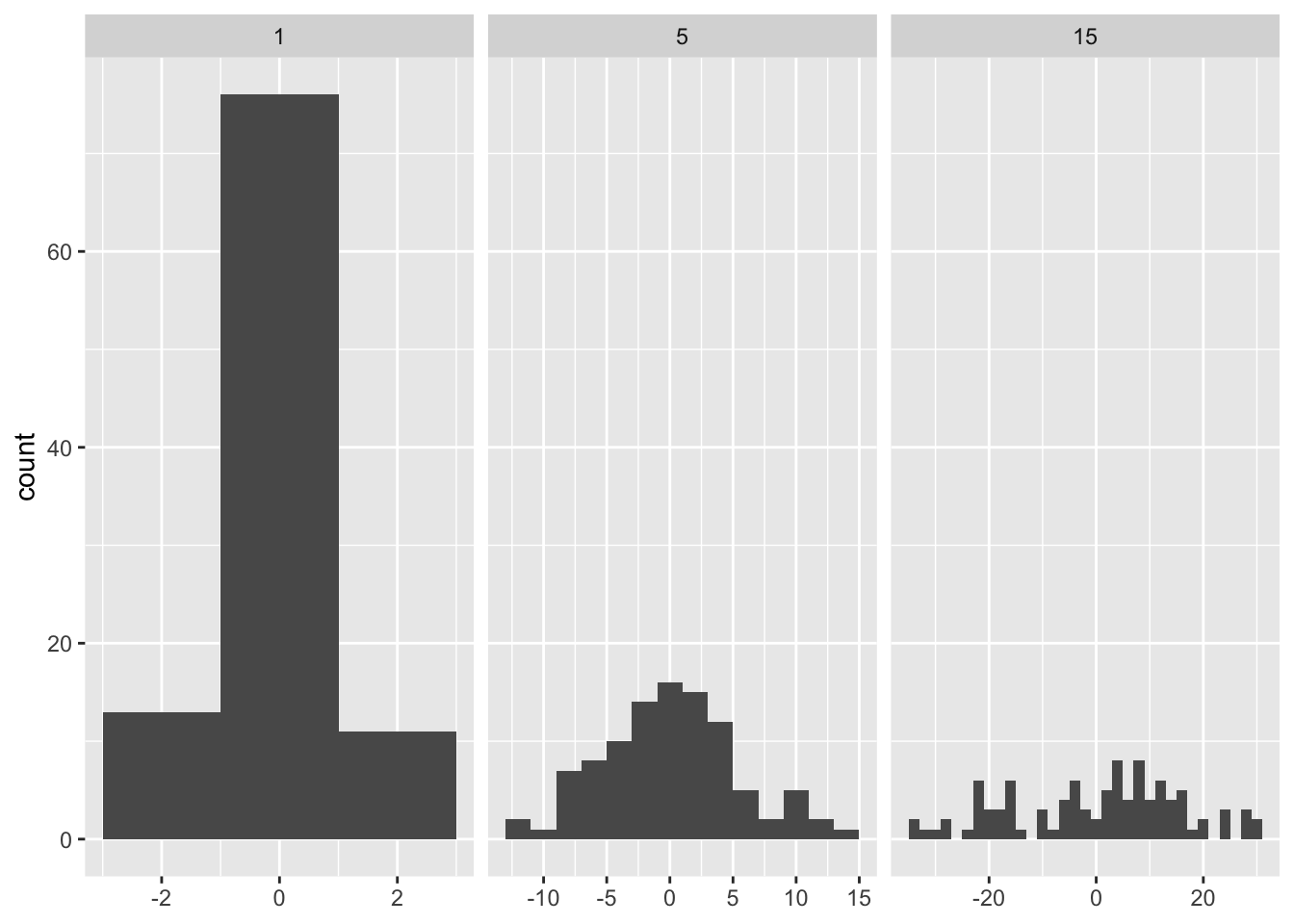In order to fix the number of bins per facet we can pass a function instead.

binwidth_bins <- function(n) {
force(n)

function(x) {
(max(x) - min(x)) / n
}
}

ggplot(df, aes(x)) +
geom_histogram(binwidth = binwidth_bins(20)) +
facet_wrap(~ sd, scales = "free_x") +
labs(x = NULL)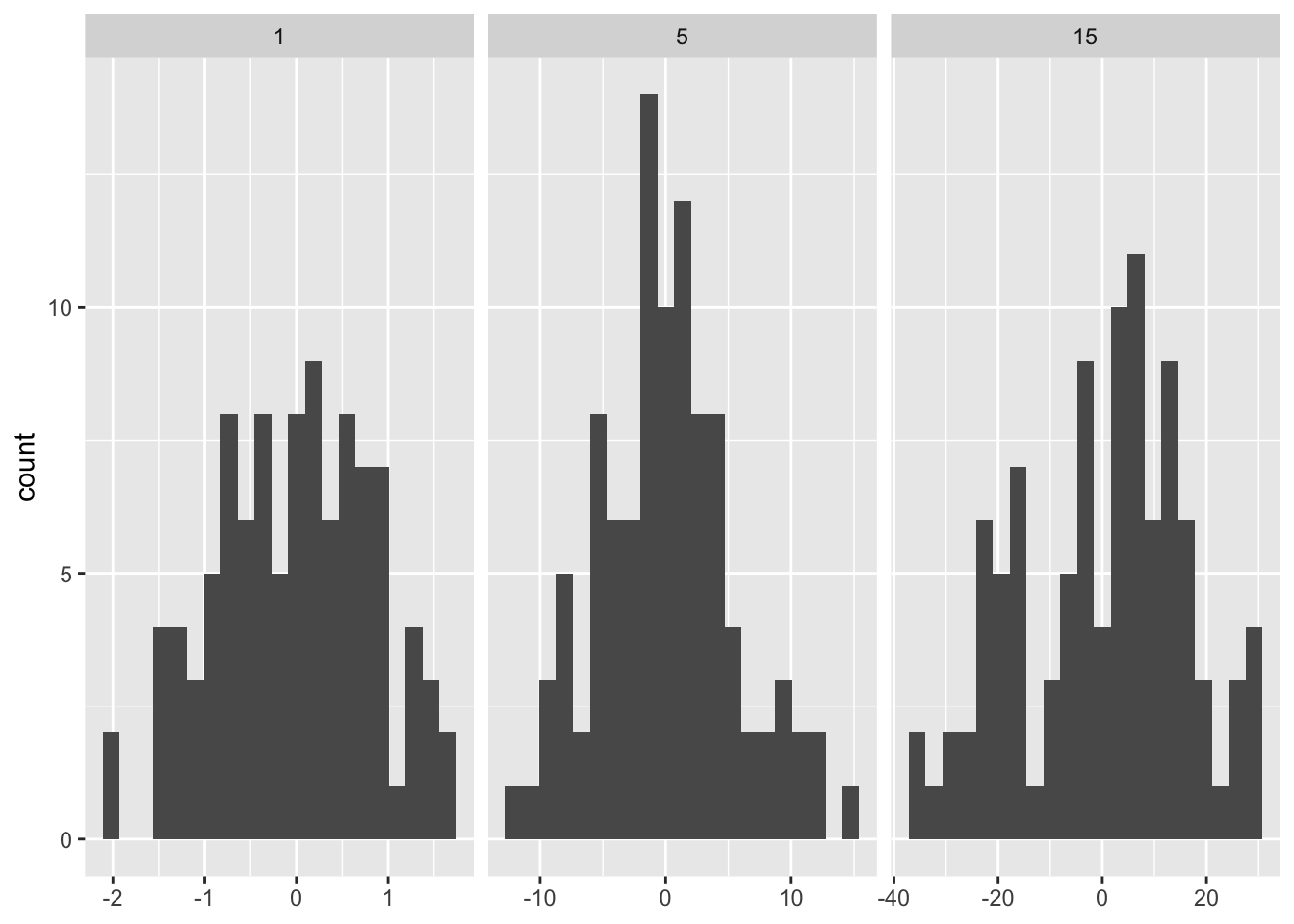geom_function() and stat_function()

in ggplot2 stat_function() and geom_function() allow you to plot a function that returns y of a given x values.

Here the density derived from 100 samples from a normal distribution vs the actual normal density function.

ggplot(data.frame(x = rnorm(100)), aes(x)) +
geom_density() +
geom_function(fun = dnorm, colour = "red")or using statfunction()

ggplot(data.frame(x = rnorm(100)), aes(x)) +
geom_density() +
stat_function(fun = dnorm, geom = "line", colour = "red")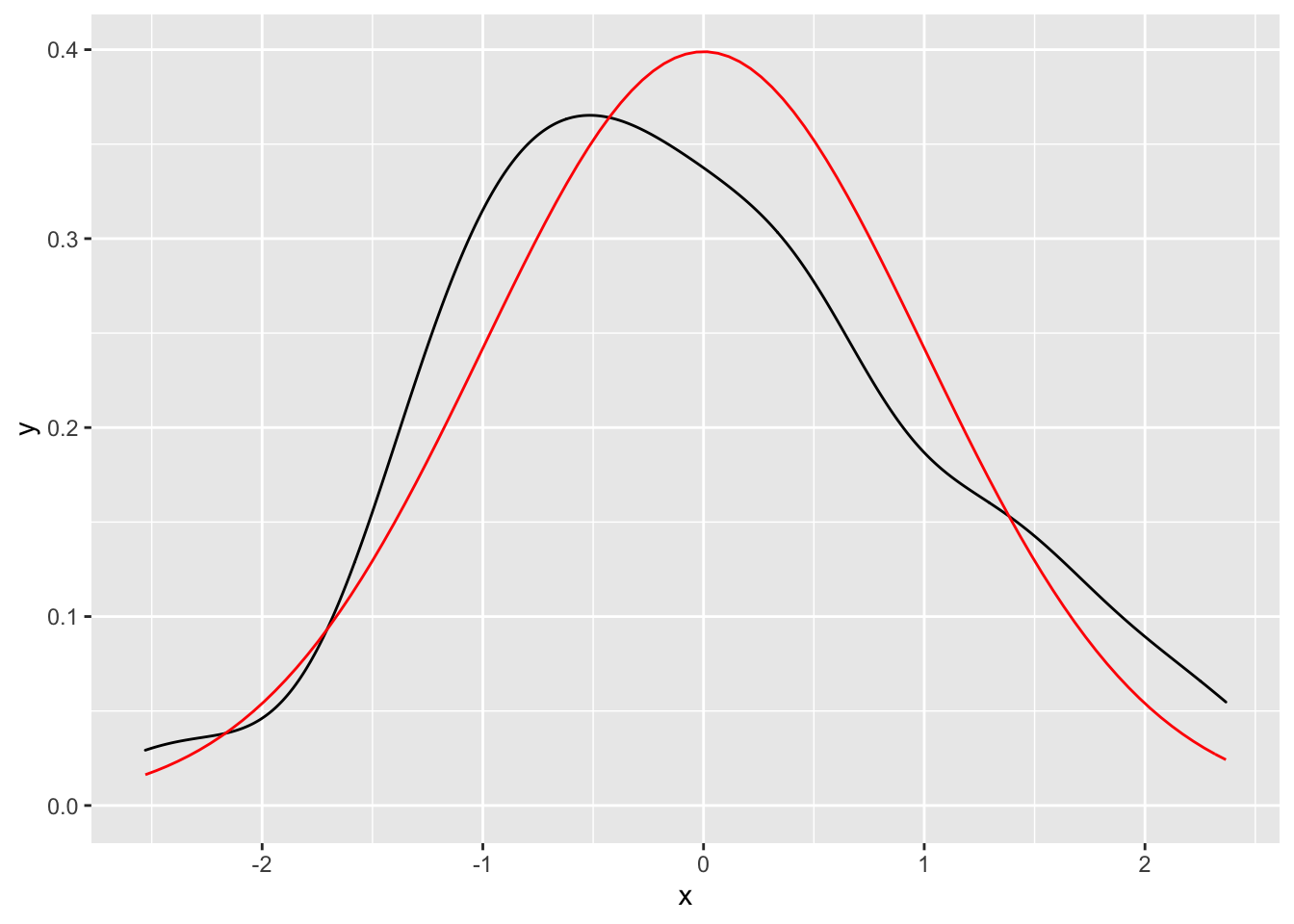function only

ggplot() +
stat_function(fun = dnorm, geom = "line", colour = "red") +
lims(x = c(-3, +3))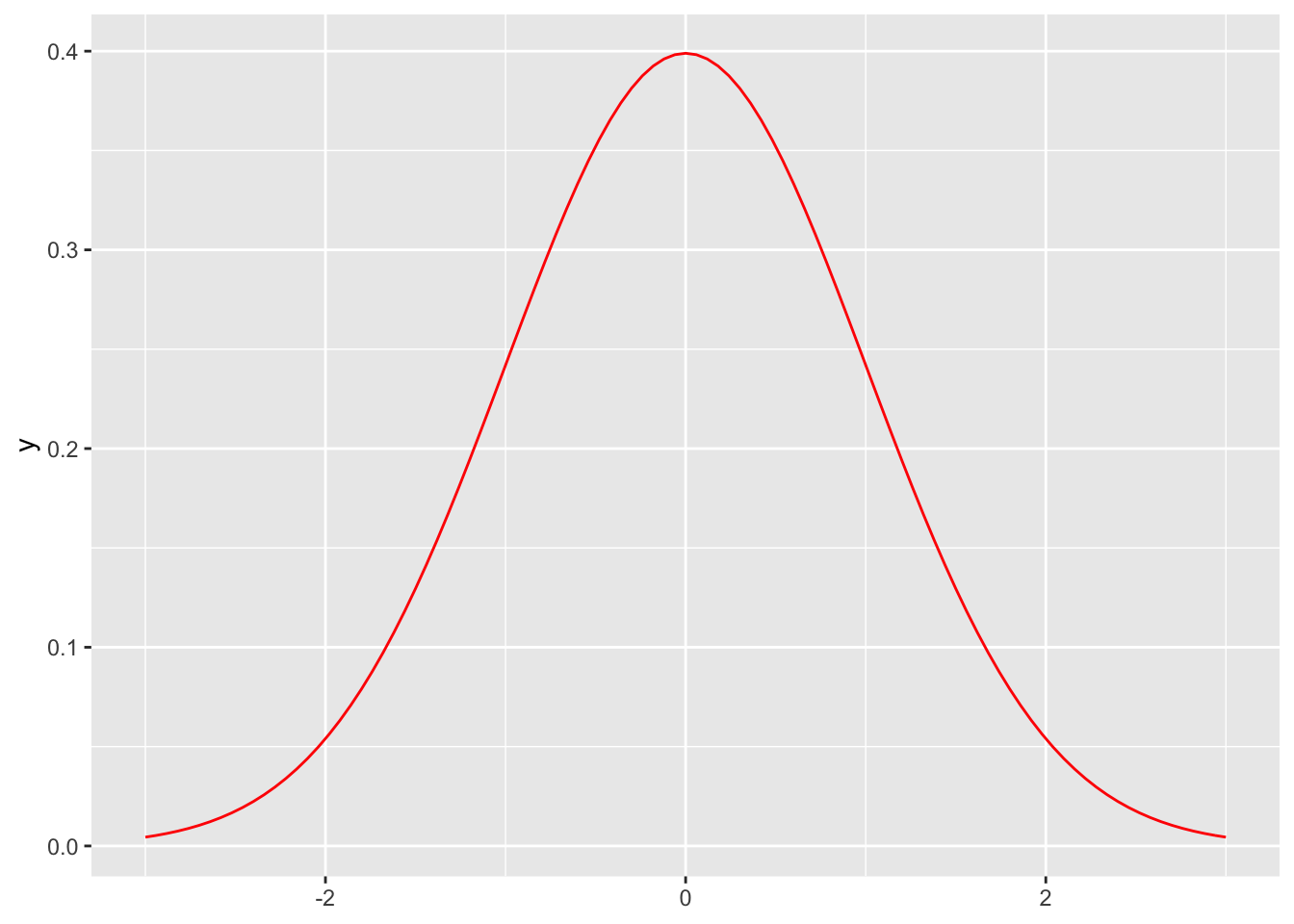Boxcox Transformation

boxcox transformation is a power transformation that is used to convert non normal distributed values to a normal distribution. The degree of the transformation is defined by an unspecified lambda. For lambda == 0 it results in a log(x) transformation.

boxcox1 <- function(x, lambda) {
if (lambda == 0) {
log(x)
} else {
(x ^ lambda - 1) / lambda
}
}

in order to plot boxcox transformations for different lambdas we need to generate a function that takes only one x argument.

boxcox2 <- function(lambda) {
if (lambda == 0) {
function(x) log(x)
} else {
function(x) (x ^ lambda - 1) / lambda
}
}

ggplot() +
geom_function(aes(colour = 0), fun = boxcox2(0)) +
geom_function(aes(colour = 1), fun = boxcox2(1)) +
geom_function(aes(colour = 1.5), fun = boxcox2(1.5)) +
lims(x = c(0, 10))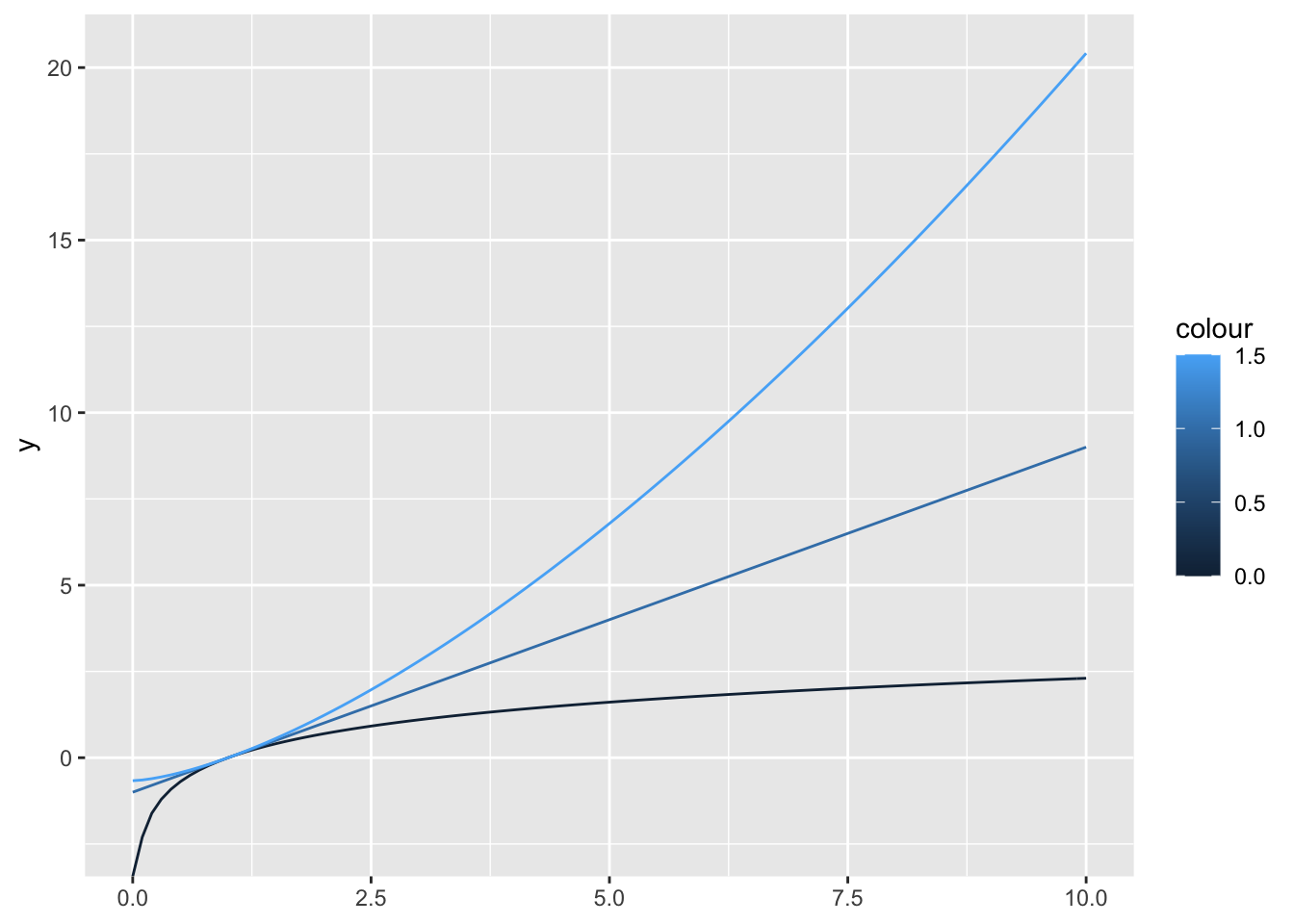we can wrap the geom calls into another function and use lapply()

geoms_boxcox <- function(lambda, ...) {
geom_function(aes(colour = lambda), fun = boxcox2(lambda), ...)
}

ggplot() +
lapply(c(0, 1, 1.5), geoms_boxcox) +
lims(x = c(0, 10))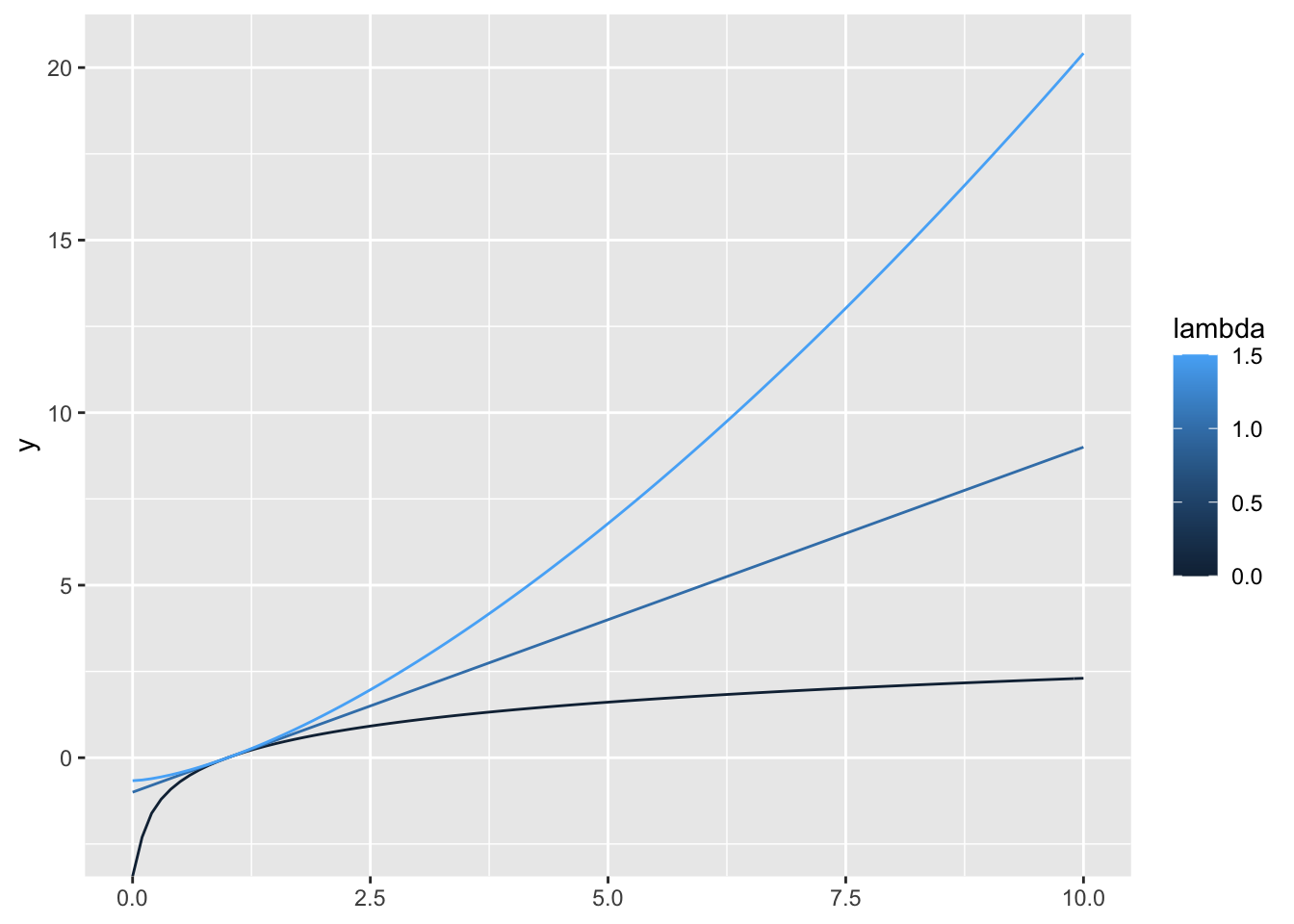Bootstraping

bootstrap resampling to permute columns in a dataframe

boot_permute <- function(df, var) {
n <- nrow(df)
force(var)

function() {
col <- df[[var]]
col[sample(n, replace = TRUE)]
}
}

boot_mtcars1 <- boot_permute(mtcars, "mpg")
##  32.4 32.4 24.4 33.9 21.4 21.5
##  26.0 13.3 15.0 17.3 15.2 15.5

Imagine we would want to resample residuals of a model in order to bootstrap error statistics

mod <- lm(mpg ~ wt + disp, data = mtcars)
# fitted returns fitted values, same as predict without newdata argument
fitted <- unname(fitted(mod))
pred <- unname(predict(mod))
stopifnot(identical(round(fitted, 6), round(pred, 6)))

resid <- unname(resid(mod))
##  -2.345433 -1.490972 -2.472367  1.785333  1.647193 -1.278631
boot_resid <- boot_permute(tibble(resid = resid), "resid")
##  -2.472367 -3.408680  6.348440  2.192792 -2.862147 -2.317052
##   1.3973583 -2.1005656  0.8901898 -0.2734911 -2.4723669 -2.0291802

we can refactor, and remove the model fitting into the factory.

boot_model <- function(df, formula) {
mod <- lm(formula, data = df)
resid <- unname(resid(mod))
# remove the model to save memory
rm(mod)

function() {
sample(resid, size = length(resid), replace = TRUE)
}
}

boot_mtcars2 <- boot_model(mtcars, mpg ~ wt + disp)
##   2.728799  2.192792  2.728799  6.348440 -2.317200 -1.278631
##  -2.47236688 -0.03421046  0.89018976  2.19486787 -2.31719966  0.22354778

Maximum Likelihod Estimation

find the parameter of a distribution that fits some observed values

lprob_poisson <- function(lambda, x) {
n <- length(x)
(log(lambda) * sum(x)) - (n * lambda) - sum(lfactorial(x))
}

obs <- c(41, 30, 31, 38, 29, 24, 30, 29, 31, 38)

lprob_poisson(10, obs)
##  -183.6405
lprob_poisson(20, obs)
##  -61.14028
lprob_poisson(30, obs)
##  -30.98598

the term sum(lfactorial(x) is independent of lambda and can be precomputed to optimise the calculation

c <- sum(lfactorial(x))

lprob_poisson1 <- function(lambda, x, c) {
n <- length(x)
(log(lambda) * sum(x)) - (n * lambda) - c
}

lprob_poisson(10, obs)
##  -183.6405

we can also move x and c into the function

lprob_poisson2 <- function(x) {

# we do not need to force(x)
# since it is not used by the
# returned function
n <-length(x)
c <- sum(lfactorial(x))
sum_x <- sum(x)

function(lambda) {
(log(lambda) * sum_x) - (n * lambda) - c
}
}

ll1 <- lprob_poisson2(obs)
ll1(10)
##  -183.6405

we can use optimise to find the best value

optimise(f = ll1, interval = c(0, 100), maximum = TRUE)
## \$maximum
##  32.09999
##
## \$objective
##  -30.26755

Function Operators

Functions that take a function as an argument and return a modfified function. Are called decorators in python.

• purrr::safely() and friends
• memoise::memoise() (see below)
• any reusable wrapper, for example one that automatically retries a give function on failure

memoise::memoise()

caching the results of a slow function when executed again with the same arguments

slow_function <- function(x) {
Sys.sleep(1)
x * 10 * runif(1)
}

# different results
system.time(print(slow_function(1)))
##  3.095154
##    user  system elapsed
##   0.001   0.000   1.004
system.time(print(slow_function(1)))
##  9.585923
##    user  system elapsed
##   0.004   0.000   1.008
fast_function <- memoise::memoise(slow_function)

# same results because cached result is returned
system.time(print(fast_function(1)))
##  1.817053
##    user  system elapsed
##   0.001   0.000   1.003
system.time(print(fast_function(1)))
##  1.817053
##    user  system elapsed
##   0.022   0.000   0.022
# different result because argument has changed
system.time(print(fast_function(2)))
##  6.450071
##    user  system elapsed
##       0       0       1

Recursion

a classical example for recursive functions is the fibinacci sequence

fib <- function(n) {
if (n < 2) return(1)
fib(n - 2) + fib(n - 1)
}
system.time(print(fib(23)))
##  46368
##    user  system elapsed
##   0.050   0.002   0.052
system.time(print(fib(33)))
##  5702887
##    user  system elapsed
##   5.056   0.050   5.138

memoise::memoise() will cache the results

fib2 <- memoise::memoise(function(n) {
if (n < 2) return(1)
fib2(n - 2) + fib2(n - 1)
})

system.time(fib2(23))
##    user  system elapsed
##   0.009   0.000   0.010
system.time(fib2(33))
##    user  system elapsed
##   0.003   0.000   0.003

OOP

• encapsulated OOP object.method(arg1, arg2)
• functional OOP generic(object, arg1, arg2)

S3

Functional OOP framework that is most commonly used in R.

There are very few constrictions for creating S3 classes but some conventions.

• create a low-level constructor that creates objects with the right structure called new_myclass()
• a validator that performs computationally expensive checks, called validate_myclass()
• a user-friendly helper called myclass()

simaerep example

the simaerep v0.3.1 package currently performs a sequence of operations on a dataframe. Each operations requires a different function that takes a data.frame and returns a modified version. We could simplify the interface for the users by grouping all those operations using S3 classes.

There is no function wrapping the entire workflow because we want intermediary results to be accessible and visible. By convention a function returns only one object which would then only return the final dataframe df_eval. Then we would loose df_site and df_sim_sites for plotting and inspection.

• simplified user interface
• input checks only need to run once
• preserve intermediary results as class attributes
# devtools::install_github("openpharma/simaerep@v0.3.1")

suppressPackageStartupMessages(library("simaerep"))

set.seed(1)

df_visit <- sim_test_data_study(
n_pat = 1000, # number of patients in study
n_sites = 100, # number of sites in study
frac_site_with_ur = 0.05, # fraction of sites under-reporting
ur_rate = 0.4, # rate of under-reporting
ae_per_visit_mean = 0.5 # mean AE per patient visit
)

df_visit\$study_id <- "A"

df_site <- site_aggr(df_visit)

df_sim_sites <- sim_sites(df_site, df_visit, r = 1000)

df_eval <- eval_sites(df_sim_sites)

plot_study(df_visit, df_site, df_eval, study = "A")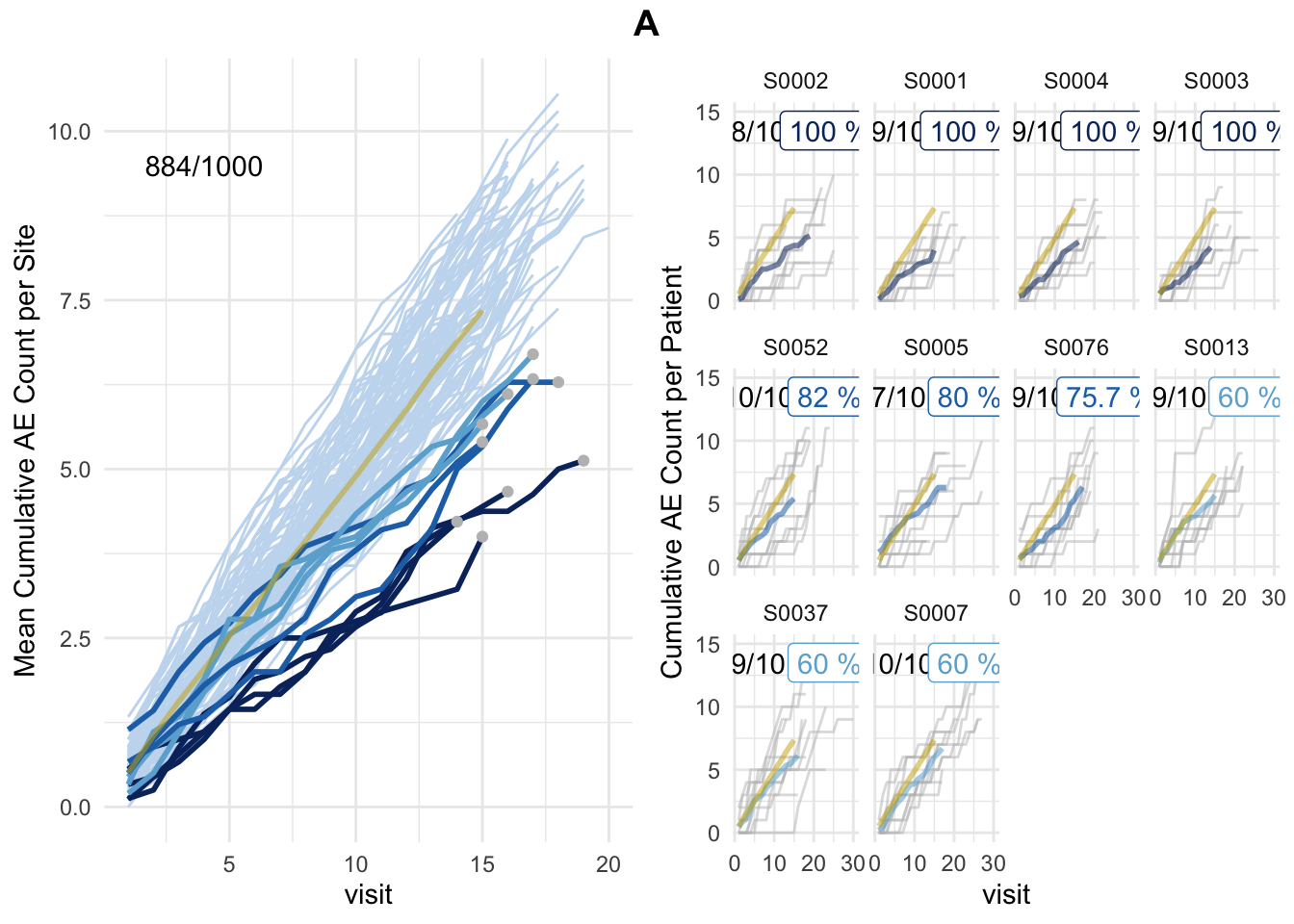constructor

new_simaerep <- function(df_site,
df_sim_sites,
df_eval,
r) {

structure(
list(
df_site = df_site,
df_sim_sites = df_sim_sites,
df_eval = df_eval,
r = r
),
class = "simaerep"
)
}

validator

validate_simaerep <- function(obj) {
comp <- sort(attributes(obj)\$names) ==  sort(c("df_site", "df_sim_sites", "df_eval", "r"))
stopifnot(all(comp))
return(obj)
}

user-friendly constructor

simaerep <- function(df_visit, r = 1000) {

df_site <- site_aggr(df_visit)

df_sim_sites <- sim_sites(df_site, df_visit, r = 1000)

df_eval <- eval_sites(df_sim_sites)

validate_simaerep(
new_simaerep(
df_site,
df_sim_sites,
df_eval,
r
)
)
}

simaerep_studyA <- simaerep(df_visit)

str(simaerep_studyA)
## List of 4
##  \$ df_site     : tibble [100 × 6] (S3: tbl_df/tbl/data.frame)
##   ..\$ study_id          : chr [1:100] "A" "A" "A" "A" ...
##   ..\$ site_number       : chr [1:100] "S0001" "S0002" "S0003" "S0004" ...
##   ..\$ n_pat             : int [1:100] 10 10 10 10 10 10 10 10 10 10 ...
##   ..\$ n_pat_with_med75  : int [1:100] 9 8 9 9 7 8 10 7 10 8 ...
##   ..\$ visit_med75       : Named num [1:100] 15 19 14 16 18 19 17 16 16 16 ...
##   .. ..- attr(*, "names")= chr [1:100] "80%" "80%" "80%" "80%" ...
##   ..\$ mean_ae_site_med75: num [1:100] 4 5.12 4.22 4.67 6.29 ...
##  \$ df_sim_sites: tibble [100 × 9] (S3: tbl_df/tbl/data.frame)
##   ..\$ study_id           : chr [1:100] "A" "A" "A" "A" ...
##   ..\$ site_number        : chr [1:100] "S0001" "S0002" "S0003" "S0004" ...
##   ..\$ n_pat              : int [1:100] 10 10 10 10 10 10 10 10 10 10 ...
##   ..\$ n_pat_with_med75   : int [1:100] 9 8 9 9 7 8 10 7 10 8 ...
##   ..\$ visit_med75        : Named num [1:100] 15 19 14 16 18 19 17 16 16 16 ...
##   .. ..- attr(*, "names")= chr [1:100] "80%" "80%" "80%" "80%" ...
##   ..\$ mean_ae_site_med75 : num [1:100] 4 5.12 4.22 4.67 6.29 ...
##   ..\$ mean_ae_study_med75: num [1:100] 7.38 9.33 6.92 7.91 8.84 ...
##   ..\$ pval               : num [1:100] 7.14e-05 3.15e-05 1.38e-03 2.60e-04 2.11e-02 ...
##   ..\$ prob_low           : num [1:100] 0 0 0 0 0.012 0.431 0.04 1 1 0.395 ...
##  \$ df_eval     : tibble [100 × 13] (S3: tbl_df/tbl/data.frame)
##   ..\$ study_id           : chr [1:100] "A" "A" "A" "A" ...
##   ..\$ site_number        : chr [1:100] "S0002" "S0001" "S0004" "S0003" ...
##   ..\$ n_pat              : int [1:100] 10 10 10 10 10 10 10 10 10 10 ...
##   ..\$ n_pat_with_med75   : int [1:100] 8 9 9 9 10 7 9 9 9 10 ...
##   ..\$ visit_med75        : Named num [1:100] 19 15 16 14 15 18 17 15 16 17 ...
##   .. ..- attr(*, "names")= chr [1:100] "80%" "80%" "80%" "80%" ...
##   ..\$ mean_ae_site_med75 : num [1:100] 5.12 4 4.67 4.22 5.4 ...
##   ..\$ mean_ae_study_med75: num [1:100] 9.33 7.38 7.91 6.92 7.37 ...
##   ..\$ pval               : num [1:100] 3.15e-05 7.14e-05 2.60e-04 1.38e-03 2.19e-02 ...
##   ..\$ prob_low           : num [1:100] 0 0 0 0 0.009 0.012 0.017 0.036 0.038 0.04 ...
##   ..\$ pval_adj           : num [1:100] 0.00315 0.00357 0.00867 0.03444 0.36483 ...
##   ..\$ pval_prob_ur       : num [1:100] 0.997 0.996 0.991 0.966 0.635 ...
##   ..\$ prob_low_adj       : num [1:100] 0 0 0 0 0.18 ...
##   ..\$ prob_low_prob_ur   : num [1:100] 1 1 1 1 0.82 ...
##  \$ r           : num 1000
##  - attr(*, "class")= chr "simaerep"

adding some class methods for common generic functions like print(), plot()

print.simaerep <- function(obj) {
studies <- unique(obj\$df_site\$study_id)
print(paste("AE underreporting for studies:", studies))
}

print(simaerep_studyA)
##  "AE underreporting for studies: A"
plot.simaerep <- function(obj, df_visit, study, what = c("study", "med75")) {

stopifnot(study %in% unique(df_visit\$study_id))

what <- match.arg(what)
.f <- switch(
what,
study = function(...) simaerep::plot_study(..., df_eval = obj\$df_eval, study = study),
med75 = function(...) simaerep::plot_visit_med75(..., study_id_str = study)
)

.f(df_visit = df_visit, df_site = obj\$df_site)
}

plot(simaerep_studyA, df_visit, study = "A", what = "study")plot(simaerep_studyA, df_visit, study = "A", what = "med75")
## purple line:          mean site ae of patients with visit_med75
## grey line:            patient included
## black dashed line:    patient excluded
## dotted vertical line: visit_med75, 0.75 x median of maximum patient visits of site
## solid vertical line:  visit_med75 adjusted, increased to minimum maximum patient visit of included patients
## dashed vertical line: maximum value for visit_med75 adjusted, 80% quantile of maximum patient visits of study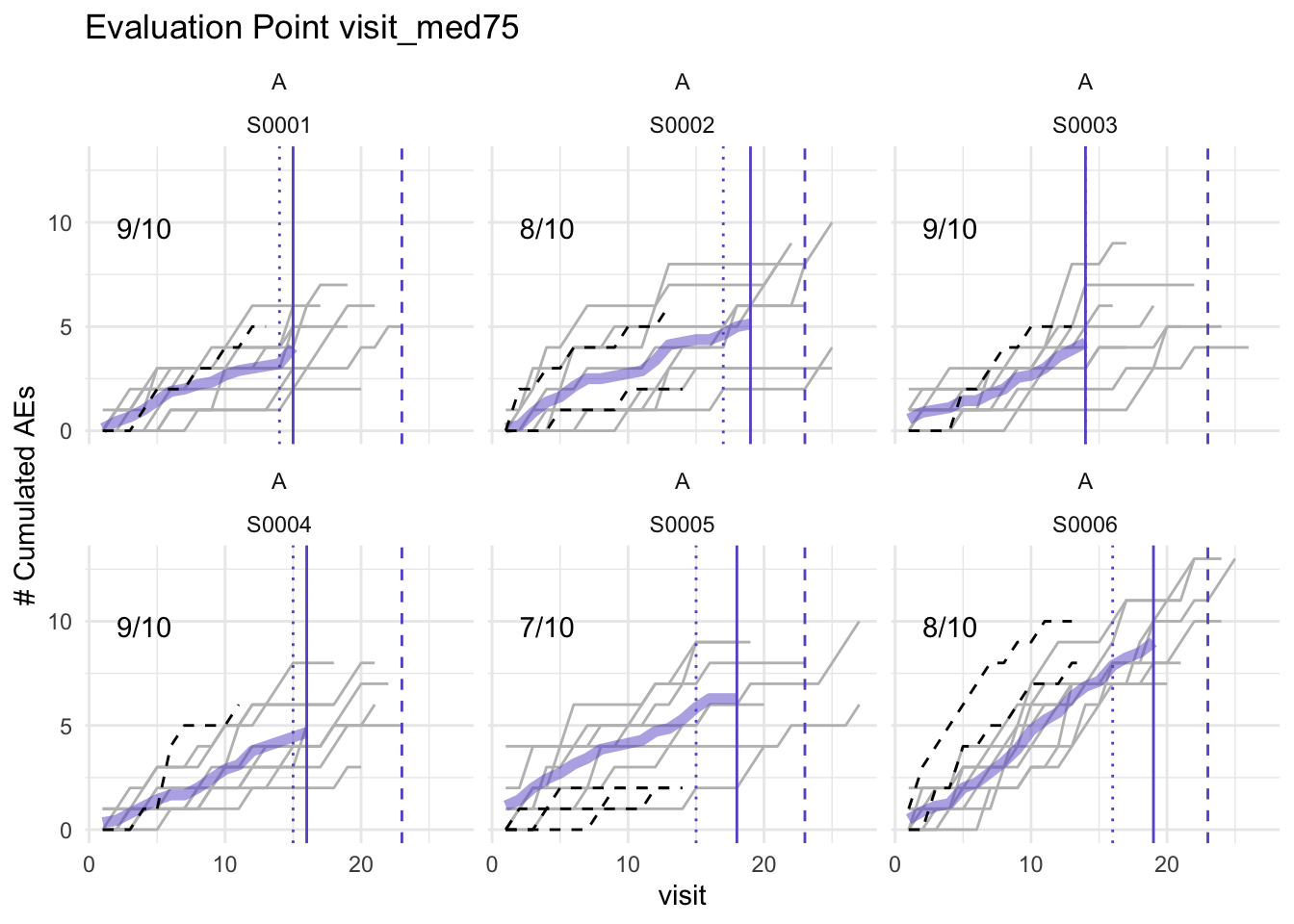to this point we have not:

• created new generic functions
• used class inheritance

A class that inherits from another class mus carry all its parents attributes, we can implement this by modifying the parent constructor as below and by calling the parent constructor from within the child constructor.

Here we will create two classes one for initiating a simaerep object and the initial df_site aggregation step and one for the simulation.

new_simaerep <- function(df_site, ..., class = character()) {
structure(
list(
df_site = df_site,
...
),
class = c(class, "simaerep")
)
}

validate_simaerep <- function(obj) {
comp <- attributes(obj)\$names ==  "df_site"
stopifnot(all(comp))
return(obj)
}

simaerep <- function(df_visit) {

df_site <- site_aggr(df_visit)

validate_simaerep(
new_simaerep(
df_site
)
)
}

simaerep_studyA <- simaerep(df_visit)

str(simaerep_studyA)
## List of 1
##  \$ df_site: tibble [100 × 6] (S3: tbl_df/tbl/data.frame)
##   ..\$ study_id          : chr [1:100] "A" "A" "A" "A" ...
##   ..\$ site_number       : chr [1:100] "S0001" "S0002" "S0003" "S0004" ...
##   ..\$ n_pat             : int [1:100] 10 10 10 10 10 10 10 10 10 10 ...
##   ..\$ n_pat_with_med75  : int [1:100] 9 8 9 9 7 8 10 7 10 8 ...
##   ..\$ visit_med75       : Named num [1:100] 15 19 14 16 18 19 17 16 16 16 ...
##   .. ..- attr(*, "names")= chr [1:100] "80%" "80%" "80%" "80%" ...
##   ..\$ mean_ae_site_med75: num [1:100] 4 5.12 4.22 4.67 6.29 ...
##  - attr(*, "class")= chr "simaerep"
new_simaerep_sim <- function(df_site, df_sim_sites, df_eval, r) {

new_simaerep(
df_site = df_site,
df_sim_sites = df_sim_sites,
df_eval = df_eval,
r = r,
class = "simaerep_sim"
)

}

validate_simaerep_sim <- function(obj) {

comp <- sort(attributes(obj)\$names) ==  sort(c("df_site", "df_sim_sites", "df_eval", "r"))
stopifnot(all(comp))
return(obj)
}

next we define a new generic method called sim that starts the simulation which returns the new class simaerep_sim. The process that finds the right method to call for an object called with a generic function is called method dispatching.

# define generic function
sim <- function(obj, ...) {
UseMethod("sim")
}

sim.simaerep <- function(obj, df_visit, r = 1000) {

df_sim_sites <- sim_sites(obj\$df_site, df_visit, r = r)

df_eval <- eval_sites(df_sim_sites)

validate_simaerep_sim(
new_simaerep_sim(
df_site,
df_sim_sites,
df_eval,
r
)
)
}

simaerep_studyA_sim <- sim(simaerep_studyA, df_visit)

str(simaerep_studyA_sim)
## List of 4
##  \$ df_site     : tibble [100 × 6] (S3: tbl_df/tbl/data.frame)
##   ..\$ study_id          : chr [1:100] "A" "A" "A" "A" ...
##   ..\$ site_number       : chr [1:100] "S0001" "S0002" "S0003" "S0004" ...
##   ..\$ n_pat             : int [1:100] 10 10 10 10 10 10 10 10 10 10 ...
##   ..\$ n_pat_with_med75  : int [1:100] 9 8 9 9 7 8 10 7 10 8 ...
##   ..\$ visit_med75       : Named num [1:100] 15 19 14 16 18 19 17 16 16 16 ...
##   .. ..- attr(*, "names")= chr [1:100] "80%" "80%" "80%" "80%" ...
##   ..\$ mean_ae_site_med75: num [1:100] 4 5.12 4.22 4.67 6.29 ...
##  \$ df_sim_sites: tibble [100 × 9] (S3: tbl_df/tbl/data.frame)
##   ..\$ study_id           : chr [1:100] "A" "A" "A" "A" ...
##   ..\$ site_number        : chr [1:100] "S0001" "S0002" "S0003" "S0004" ...
##   ..\$ n_pat              : int [1:100] 10 10 10 10 10 10 10 10 10 10 ...
##   ..\$ n_pat_with_med75   : int [1:100] 9 8 9 9 7 8 10 7 10 8 ...
##   ..\$ visit_med75        : Named num [1:100] 15 19 14 16 18 19 17 16 16 16 ...
##   .. ..- attr(*, "names")= chr [1:100] "80%" "80%" "80%" "80%" ...
##   ..\$ mean_ae_site_med75 : num [1:100] 4 5.12 4.22 4.67 6.29 ...
##   ..\$ mean_ae_study_med75: num [1:100] 7.38 9.33 6.92 7.91 8.84 ...
##   ..\$ pval               : num [1:100] 7.14e-05 3.15e-05 1.38e-03 2.60e-04 2.11e-02 ...
##   ..\$ prob_low           : num [1:100] 0 0 0 0 0.012 0.431 0.04 1 1 0.395 ...
##  \$ df_eval     : tibble [100 × 13] (S3: tbl_df/tbl/data.frame)
##   ..\$ study_id           : chr [1:100] "A" "A" "A" "A" ...
##   ..\$ site_number        : chr [1:100] "S0002" "S0001" "S0004" "S0003" ...
##   ..\$ n_pat              : int [1:100] 10 10 10 10 10 10 10 10 10 10 ...
##   ..\$ n_pat_with_med75   : int [1:100] 8 9 9 9 10 7 9 9 9 10 ...
##   ..\$ visit_med75        : Named num [1:100] 19 15 16 14 15 18 17 15 16 17 ...
##   .. ..- attr(*, "names")= chr [1:100] "80%" "80%" "80%" "80%" ...
##   ..\$ mean_ae_site_med75 : num [1:100] 5.12 4 4.67 4.22 5.4 ...
##   ..\$ mean_ae_study_med75: num [1:100] 9.33 7.38 7.91 6.92 7.37 ...
##   ..\$ pval               : num [1:100] 3.15e-05 7.14e-05 2.60e-04 1.38e-03 2.19e-02 ...
##   ..\$ prob_low           : num [1:100] 0 0 0 0 0.009 0.012 0.017 0.036 0.038 0.04 ...
##   ..\$ pval_adj           : num [1:100] 0.00315 0.00357 0.00867 0.03444 0.36483 ...
##   ..\$ pval_prob_ur       : num [1:100] 0.997 0.996 0.991 0.966 0.635 ...
##   ..\$ prob_low_adj       : num [1:100] 0 0 0 0 0.18 ...
##   ..\$ prob_low_prob_ur   : num [1:100] 1 1 1 1 0.82 ...
##  \$ r           : num 1000
##  - attr(*, "class")= chr [1:2] "simaerep_sim" "simaerep"
class(simaerep_studyA_sim)
##  "simaerep_sim" "simaerep"
plot.simaerep <- function(obj, df_visit, study,
... # we need ... to ignore additional arguments when called with NextMethod()
) {
simaerep::plot_visit_med75(df_visit, obj\$df_site, study_id_str = study)
}

override plot method of parent class, and use user-supplied argument to switch back to parent class method using NextMethod()

plot.simaerep_sim <- function(obj, df_visit, study, what = c("study", "med75")) {
what <- match.arg(what)
if (what == "study") {
simaerep::plot_study(df_visit, obj\$df_site, obj\$df_eval, study = study)
} else {
# calls parent class method using all original arguments
NextMethod()
}
}

plot(simaerep_studyA_sim, df_visit, study = "A", what = "study")plot(simaerep_studyA_sim, df_visit, study = "A", what = "med75")
## purple line:          mean site ae of patients with visit_med75
## grey line:            patient included
## black dashed line:    patient excluded
## dotted vertical line: visit_med75, 0.75 x median of maximum patient visits of site
## solid vertical line:  visit_med75 adjusted, increased to minimum maximum patient visit of included patients
## dashed vertical line: maximum value for visit_med75 adjusted, 80% quantile of maximum patient visits of studyplot(simaerep_studyA_sim, df_visit, study = "A", what = "XXX")
## Error in match.arg(what): 'arg' should be one of "study", "med75"
plot(simaerep_studyA, df_visit, study = "A")
## purple line:          mean site ae of patients with visit_med75
## grey line:            patient included
## black dashed line:    patient excluded
## dotted vertical line: visit_med75, 0.75 x median of maximum patient visits of site
## solid vertical line:  visit_med75 adjusted, increased to minimum maximum patient visit of included patients
## dashed vertical line: maximum value for visit_med75 adjusted, 80% quantile of maximum patient visits of studysloop provides some function to investigate S3 class behavior.

sloop::s3_methods_class("simaerep")
## # A tibble: 3 × 4
##   generic class    visible source
##   <chr>   <chr>    <lgl>   <chr>
## 1 plot    simaerep TRUE    .GlobalEnv
## 2 print   simaerep TRUE    .GlobalEnv
## 3 sim     simaerep TRUE    .GlobalEnv
sloop::s3_methods_class("simaerep_sim")
## # A tibble: 1 × 4
##   generic class        visible source
##   <chr>   <chr>        <lgl>   <chr>
## 1 plot    simaerep_sim TRUE    .GlobalEnv
sloop::s3_methods_generic("sim")
## # A tibble: 1 × 4
##   generic class    visible source
##   <chr>   <chr>    <lgl>   <chr>
## 1 sim     simaerep TRUE    .GlobalEnv
sloop::s3_dispatch(plot(simaerep_studyA_sim, df_visit, study = "A"))
## => plot.simaerep_sim
## -> plot.simaerep
##  * plot.default
sloop::s3_dispatch(plot(simaerep_studyA, df_visit, study = "A"))
## => plot.simaerep
##  * plot.default

R6

R6 uses the encapsulated OOP framework, commonly used in shiny. Works like object in python.

• Use R6::R6Class() to construct classes
• Pass a list with attributes (static values) and methods (functions)
• Within methods refer to the class as self and subset with \$ (self\$attribute, self.method())
• Instantiate objects by calling x <- Class\$new()
• methods can be chained x\$add(1)\$sub(1)
• initialize() method overrise new() and works like __init() in python
• inherit by using the inherit argument of R6::R6Class()
• pass private and public attributes and classes using the privat and public arguments of R6::R6Class()
• active fields look like attributes but are methods, can run additional checks
• instantiated R6 classes behave like python objects modifying one object will also modify all of its copies
• always put randomized default values that you do not want to share across instances into the initialize function

S4

Mostly used for bioconductor packages uses functional OOP framework. A well-known package that uses S4 is ROCR and lubridate

• much stricter framework than S3
• makes use of specialized functions like setClass(), setGeneric(), setMethod()
• all functions are distributed with R in the methods package (which should be explicitly loaded so show S4 framework is used and to avoid problems when running non-interactive mode)
• attributes are slots which can be subset using @
• supports multiple inheritance and multiple dispatch (which should be avoided because its complicated)

Slots

• when instantiated objects slot’s are automatically checked if they contain the correct data types
• setValidity(), validObject() can be used to extend checks and to validate existing objects

Conventions

• CamelCase
• provide constructor with same name as class
• write generics that call and set slot values

Multiple Inheritence

in case a class inherits from multiple classes the method that is closest to the class is chosen. If two methods are matching the method for the class which has a higher lexical order is chosen.

Multiple dispatch

If a generic uses two classes as input both classes are searched for methods.

S4 vs S3

• S4 more suitable for larger teams
• S4 is better with many inter related objects (for example provide methods via generics for combinations of different types of sparse matrices)